# AP Physics B : Understanding Elastic and Inelastic Collisions

## Example Questions

### Example Question #66 : Newtonian Mechanics

Ball A, traveling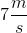to the right, collides with ball B, traveling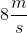to the left. If ball A is 4kg and ball B is 6kg, what will be the final velocity and direction after a perfectly inelastic collision?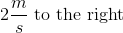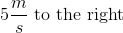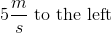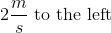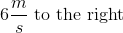Explanation:

A perfectly inelastic collision is when the two bodies stick together at the end. At the beginning the two balls are traveling separately with individual momentum values. Using the momentum equation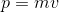, we can see that ball A has a momentum of (4kg)(7m/s) to the right and ball B has a momentum of (6kg)(8m/s) to the left. The final momentum would be the mass of both balls times the final velocity, (4+6)(vf). We can solve for vf through conservation of momentum; the sum of the initial momentum values must equal the final momentum.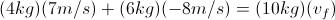Note: ball B's velocity is negative because they are traveling in opposite directions.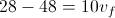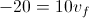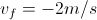The negative sign indicates the direction in which the two balls are traveling. Since the sign is negative and we indicated that traveling to the left is negative, the two balls must be traveling 2m/s to the left after the perfectly inelastic collision.